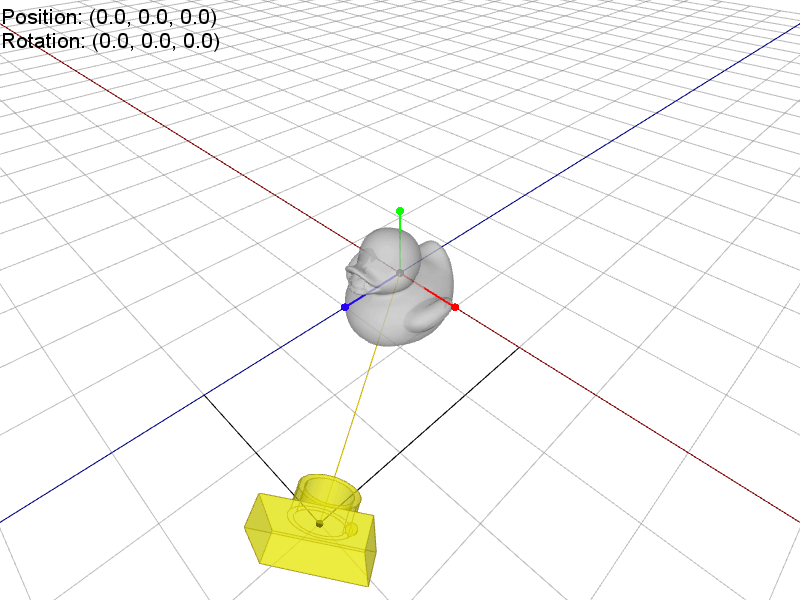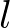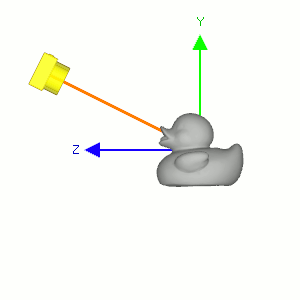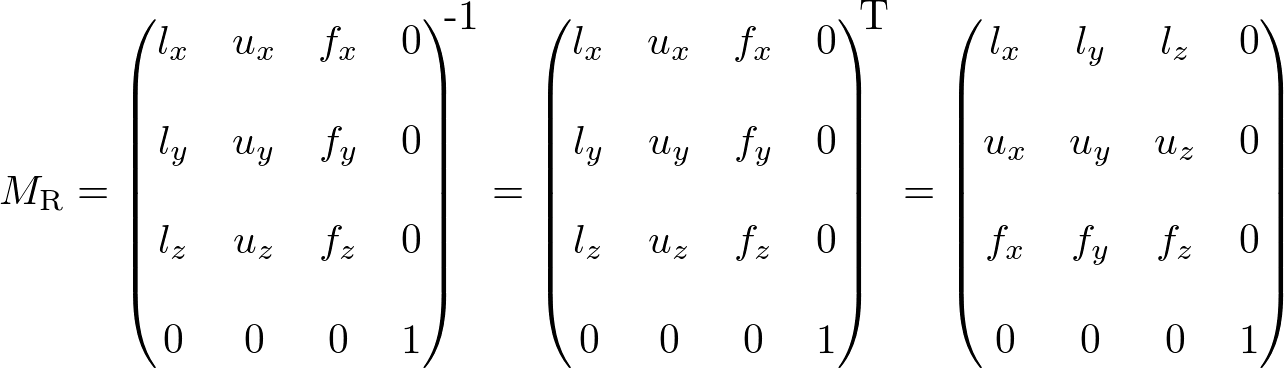# OpenGL Camera

### Under Construction...

Related Topics: OpenGL Transform, OpenGL Projection Matrix, Quaternion to Rotation Matrix

### OverviewOpenGL camera is always at origin and facing to -Z in eye space

OpenGL doesn't explicitly define neither camera object nor a specific matrix for camera transformation. Instead, OpenGL transforms the entire scene (including the camera) inversely to a space, where a fixed camera is at the origin (0,0,0) and always looking along -Z axis. This space is called eye space.

Because of this, OpenGL uses a single GL_MODELVIEW matrix for both object transformation to world space and camera (view) transformation to eye space.

You may break it down into 2 logical sub matrices;That is, each object in a scene is transformed with its own Mmodel first, then the entire scene is transformed reversely with Mview. In this page, we will discuss only Mview for camera transformation in OpenGL.

### LookAt

gluLookAt() is used to construct a viewing matrix where a camera is located at the eye positionand looking at (or rotating to) the target point. The eye position and target are defined in world space. This section describes how to implement the viewing matrix equivalent to gluLookAt().

Camera's lookAt transformation consists of 2 transformations; translating the whole scene inversely from the eye position to the origin (MT), and then rotating the scene with reverse orientation (MR), so the camera is positioned at the origin and facing to the -Z axis.OpenGL camera's lookAt() transformation

Suppose a camera is located at (2, 0, 3) and looking at (0, 0, 0) in world space. In order to construct the viewing matrix for this case, we need to translate the world to (-2, 0, -3) and rotate it about -33.7 degree along Y-axis. As a result, the virtual camera becomes facing to -Z axis at the origin.

The translation part of lookAt is easy. You simply move the camera position to the origin. The translation matrix MT would be (replacing the 4th coloumn with the negated eye position);Left, Up and Forward vectors from target to eye

The rotation part MR of lookAt is much harder than translation because you have to calculate 1st, 2nd and 3rd columns of the rotation matrix all together.

First, compute the normalized forward vectorfrom the target position vt to the eye position ve of the rotation matrix. Note that the forward vector is from the target to the eye position, not eye to target because the scene is actually rotated, not the camera is.Second, compute the normalized left vectorby performing cross product with a given camera's up vector. If the up vector is not provided, you may use (0, 1, 0) by assuming the camera is straight up to +Y axis.Finally, re-calculate the normalized up vectorby doing cross product the forward and left vectors, so all 3 vectors are orthonormal (perpendicular and unit length). Note we do not normalize the up vector because the cross product of 2 perpendicular unit vectors also produces a unit vector.These 3 basis vectors,,andare used to construct the rotation matrix MR of lookAt, however, the rotation matrix must be inverted. Suppose a camera is located above a scene. The whole scene must rotate downward inversely, so the camera is facing to -Z axis. In a similar way, if the camera is located at the left of the scene, the scene should rotate to right in order to align the camera to -Z axis. The following diagrams show why the rotation matrix must be inverted.The scene rotates downward if the camera is aboveThe scene rotates to right if the camera is at left

Therefore, the rotation part MR of lookAt is finding the rotation matrix from the target to the eye position, then invert it. And, the inverse matrix is equal to its transpose matrix because it is orthogonal which each column has unit length and perpendicular to the other column.Finally, the view matrix for camera's lookAt transform is multiplying MT and MR together;Here is C++ snippet to construct the view matrix for camera's lookAt transformation. Please see the details in OrbitCamera.cpp.

``````
// dependency: Vector3 and Matrix4
struct Vector3
{
float x;
float y;
float z;
};

class Matrix4
{
float m;
}

///////////////////////////////////////////////////////////////////////////////
// equivalent to gluLookAt()
// It returns 4x4 matrix
///////////////////////////////////////////////////////////////////////////////
Matrix4 lookAt(Vector3& eye, Vector3& target, Vector3& upDir)
{
// compute the forward vector from target to eye
Vector3 forward = eye - target;
forward.normalize();                 // make unit length

// compute the left vector
Vector3 left = upDir.cross(forward); // cross product
left.normalize();

// recompute the orthonormal up vector
Vector3 up = forward.cross(left);    // cross product

// init 4x4 matrix
Matrix4 matrix;
matrix.identity();

// set rotation part, inverse rotation matrix: M^-1 = M^T for Euclidean transform
matrix = left.x;
matrix = left.y;
matrix = left.z;
matrix = up.x;
matrix = up.y;
matrix = up.z;
matrix = forward.x;
matrix = forward.y;
matrix= forward.z;

// set translation part
matrix= -left.x * eye.x - left.y * eye.y - left.z * eye.z;
matrix= -up.x * eye.x - up.y * eye.y - up.z * eye.z;
matrix= -forward.x * eye.x - forward.y * eye.y - forward.z * eye.z;

return matrix;
}

``````

### Rotation (Pitch, Yaw, Roll)Camera Rotations; Pitch, Yaw and Roll

Other types of camera's rotations are pitch, yaw and roll rotating at the position of the camera. Pitch is rotating the camera up and down around the camera's local left axis (+X axis). Yaw is rotating left and right around the camera's local up axis (+Y axis). And, roll is rotating it around the camera's local forward axis (+Z axis).

First, you need to move the whole scene inversely from the camera's position to the origin (0,0,0), same as lookAt() function. Then, rotate the scene along the rotation axis; pitch (X), yaw (Y) and roll (Z) independently.

If multiple rotations are applied, combine them sequentially. Note that the different order of rotations produces a different result. A commonly used rotation order is roll -> yaw -> pitch. It produces a free-look camera or first-person shooter camera interface.

Keep it mind that the directions of the rotations (right-hand rule); a positive pitch angle is rotating the camera downward, a positive yaw angle means rotating the camera left, and a positive roll angle is rotating the camera clockwise.

### Example: OpenGL Orbit CameraOrbitCamera.zip (include VS 2015 project)